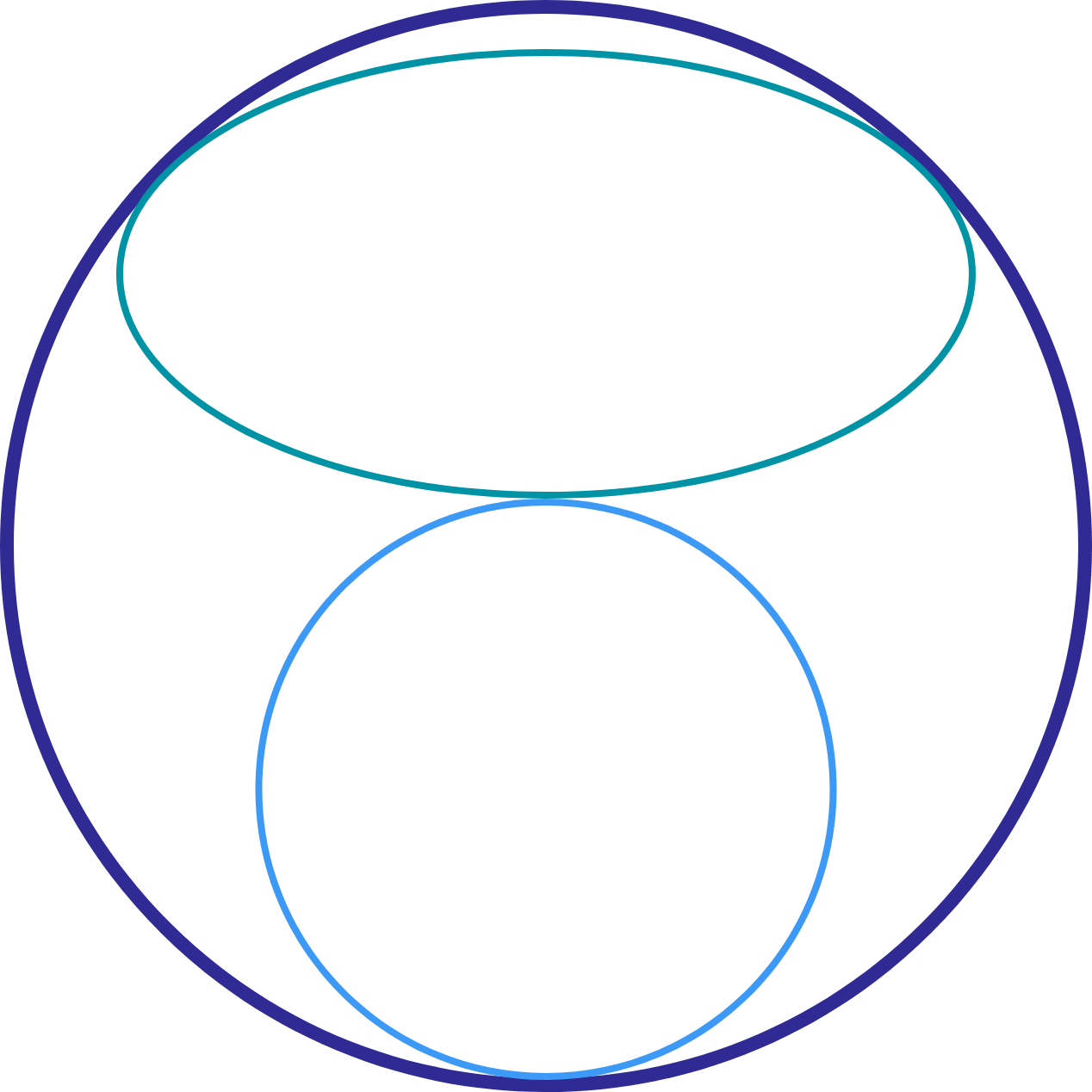# Falzoon's Ellipse

Calculus Level 3

A circle and an ellipse of the same area share the interior of a larger circle, without overlap.For the size of the smaller circle, the ellipse has the largest possible area that could fit in the space between the smaller and larger circle. Let $a$ be the combined areas of the ellipse and the small circle, and let $b$ be the area of the large circle.

Find $\left\lfloor 10000\dfrac { a }{ b } \right\rfloor$

You may want to use a computer for this.

×